# PHY3: REFLECTION

##### This unit is about the Reflection of light both in concave and convex mirrors.

REFLECTION

Reflection is the bouncing off of light rays when they strike a surface. If the surface is highly polished and smooth, reflection is said to be regular otherwise cough surface produced irregular of diffuse reflection.G – Glancing angle

AO incident ray

OB – reflected ray

O – The normal

mmL – reflecting surface

O – Point of incidence

I – point of incidence

r – Angle of reflection

g – Glancing angle

Laws of reflection

Law 1

States that the incident ray, the reflected ray and the normal at the point of incidence all lay at the same plane

Law 2

The angle of incidence is equal to the angle of reflection

An experiment to verify the laws of reflection of light

Apparatus;-

• Soft board
• A sheet of white paper
• Mirror support
• Plasticine
• 4 optical pins
• 4 drawing pins
• Plane mirror
• Mathematic instrumentsSet a strip of plane mirror along a line MM1 drawn on a sheet of white paper which is fixed on a 20ft board

A pin that serves an object is fixed into the paper 7 or 8cm from mm1. With the eye in a convenient position E1. Pins E1 and E2 are fixed into a paper so that they are in a straight line with 1 image of OC in the marrows. Pins P1 and P1 are then removed and their positions marked.

The same procedure is carried out with I in position E2 which is a on the other side of O. at least 2 such positions on the either sides of the objet should be considered. Remove all pins with ruler, draw lines through marked position of pins. Extend lined and hat they cross mm1 and to intersect at I.

The line OB1, OB2…………… represents incident rays while the [rays] lines B1 P2 B2 P4…… are the corresponding reflected rays.

Normal’s B1, N1, B2, N2 … are constructed and the of incidences

I, I, I, and of reflection R1R1 are measured and recorded in the table below;-

Within the limitations of experimental error, the angles of incidences must be equal to the angles of reflection hence verifying the 2nd law of reflection.

When a set of parallel light rays strikes a plane or a flat surface the reflected rays are also parallel: this is because the angle of incidence for each ray as it strikes the surface is the same.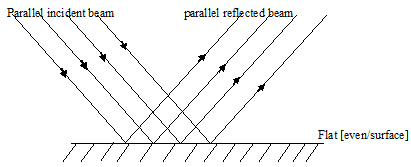When a set of parallel light rays strikes an irregular or uneven surface, such as concrete, the rays are scattered in different direction after reflection, this is so because the angle of incidence for each ray is different when they strike the uneven surface.Plane mirrors

-The image in plane mirrors is;-

-The same size as the object

-The same distance behind the mirror as the object is in front

-Laterally inverted

-Image in virtual – cannot be formed on a screen.

Types of images

1. Real images

A real image is formed when light rays from the objects actually arrive at the point, where the image is formed. These images can be obtained on a screen e.g. image in a pin hole camera or on a photographic plate.

1. Virtual image

This is an image which is formed n a plane mirror when light paths/rays appear to meet at the position of the image. The image of the mirror is due to tracing backwards the path of reflected rays from the mirror surface. The paths are usually represented by broken line.

How an image is seen in a plane mirrorThe eyes sees image due the path of the reflected ray being pressed backwards as in the above diagram.

NB:

Complete real rays F

OAE1 and OBE2

While A1 and B1 are pencil extensions which are used to trace the image of the positions

Images in two mirrors, included at 90oI1 is the image of O as a result of single reflection in M1, I2 is the image of O after a single reflection in M2 while I1, 2 is the image of O after a reflection in m1 then M2.

The number of images produced by mirrors inclined at an angle is given by the following formulae.N = the number of images

O = angle between the 2 mirrors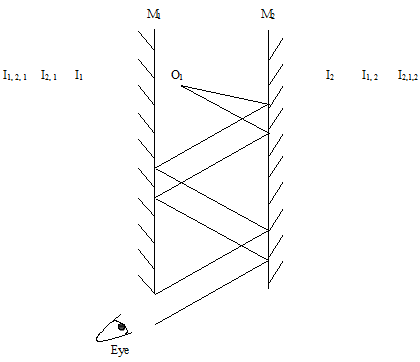Multiple images are produced by mirrors that are arranged in parallel, the further the image is from the mirrors, the fainter/ less district it appears. This is due to the subsequent less of energy of light, every time it’s reflected from the mirror surface.

I1 and I2 are images after single reflections, I1, 2, and I2, 1, I2, 1, 2, 6, I1, 2, 1 and so on are images after multiple reflections.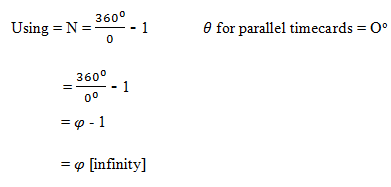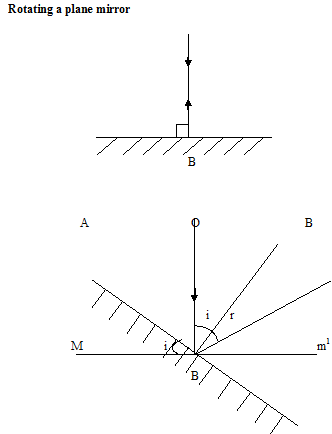In the diagram a., the incident is reflected back along its own path since it is along the incident ray mirror. When the mirror is rotated by I o as in b., and the incident ray direction maintained the normal is also rotated by I o. The reflected ray is therefore rotated through I + r o which is either equal to 2 i or 2 r- since I is equal to r.

In summary

When he mirror is turned through an angle o the reflected is turned through an angle 2q

I.e.; – 2 times [twice] the angle of rotation of the mirror

Applications

The periscopePeriscope consists of two plane mirrors fixed parallel to and facing each other in a tube. The mirrors are placed at 45o to the length of the tube.

This turns the direction of light from an object by 90o in each mirror. A periscope is used to see above an obstacle that blocks a direct deal e.g. submarines; double Decker buses so as to allow the driver see people in the upper Decker and sporting events.

Spherical [curved mirrors]

Curved mirrors can be concave or convex. Concave mirror curves inwards while convex mirror curves outside.

Common terms used for these types of mirrors

1. The pole[p]

The pole is the centre of the carved mirror

1. Center of curvature

The centre of curvature [c] is the centre of the sphere of which the mirror is part

1. Radius of curvature

Is the distance between the pole of the mirror and centre of the curvature?

1. Principle axis

Is a line through the centre of curvature and the pole of the mirror?

1. Principal focus

Is a point on the principle axis to which all rays close and parallel to the principle axis converge [concave mirror] or from which they appear to diverge [convex] after reflection from the mirror.

1. Focal length[f]

Is the distance between the pole and the principle focus?

Note

The focal length of a small mirror of the small aperture is equal to half the radius of curvature

ie;-

f = 1/2 r

Or

r = 2f

Reflection by a concave or converging mirrorThe image formed by a concave mirror is real since actual rays meet after reflection from the mirror hence the principle forces and focal length are all real.

Reflection by a convex or diverging mirror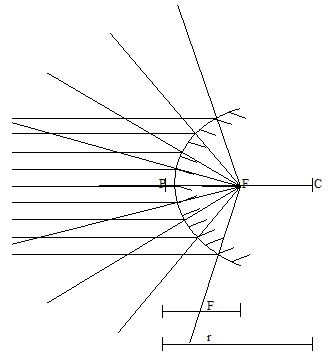The rays appear to be diverging from point [f] behind the mirror after reflection.

If a screen is placed at point F no image is formed on it thus diverging mirror has a vertical principle focus, a virtual focal length and a virtual image.

Spherical aberration

It is experienced by mirrors of large aperture where parallel rays of light are not all reflected to sharp focus but rather intersect to form a surface called a caustic.

A caustic surface is a bright curved surface that can often be seen on tea in a cup.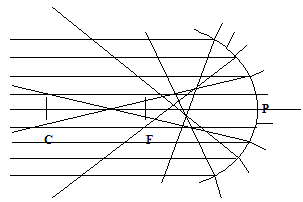Spherical aberration can be overcome by using parabolic mirrorsThe parabolic mirror has the advantages that a source placed at its principal force [f] gives rise to a beam of parallel rays.

Image formed by a concave mirror

The images formed by concave mirrors can be constructed by using ray diagrams. The following rules are used to construct these diagrams.

Note

These rules apply to only concave mirrors.

Rule 1

A ray parallel and close to the principle axis is reflected through the principle focus.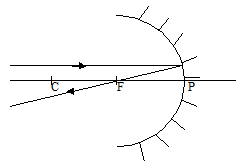Rule 2

A ray passing through the principle focus is reflected close and parallel to the principle focus.Rule 3

A ray that passes through the centre of curvature is reflected back along its own path [because it’s normal to the surface of the mirror.Rule 4

A ray that strikes the pole is reflected such that the incident ray and the reflected ray make the angle with the principle axis.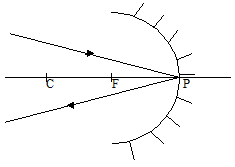Note

A real image is formed by the actual intersection of rays while a virtual image is formed when the rays appear to intersect [when their paths have been produced backwards].

Practically real image and he formed which

The any peer pays of points such that any pair posses

The principle of intervening an object and real image is called the principle of reversibility of light.

Ray diagrams for a concave mirror

Object between F and PImages

1 -Behind the mirror

2 -Upright [erect]

3 -Virtual

4-Magnified

Object at F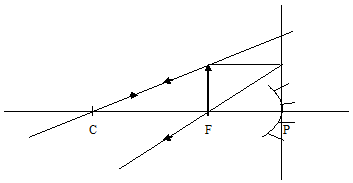Image is at infinity

Object between C and FImages

1 -behind C

2 -real

3 -inverted

4 -has been magnified

Object at CImage

1 -it is real

2 -it is inverted

3 -at C

4 -same size as objectImage

1 -real

2 -inverted

3 -diminished

4 -between C and F

Object at infinity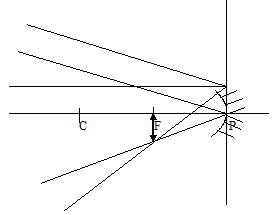Image

1 -real

2 -inverted

3 -diminished

4 -A+F

Image formation by a convex mirror

The rules of image formation and construction of ray diagrams are the same as that of concave mirrors. Since the principal focuses are virtual; all images formed by a convex mirror are always virtual, upright, behind the mirror and diminished.Magnification [m]

Magnification is the ratio of image height [size] to the object height [size].

M = Image height/ Object height

Or

Magnification is the ratio of the distance of the image from the pole to th distance of the object from the pole

M = Image distance/ Object distance

THIS VIDEO BRIEFLY EXPLAINS REFLECTION OF LIGHT

## STEM Elearning

We at FAWE have built this platform to aid learners, trainers and mentors get practical help with content, an interactive platform and tools to power their teaching and learning of STEM subjects, more

#### How to find your voice as a woman in Africa

© FAWE, Powered by: Yaaka DN.